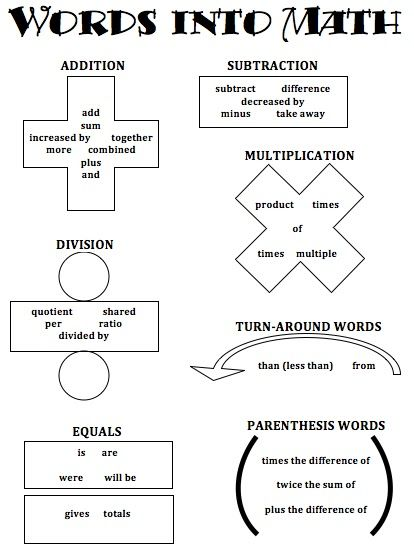# Order of Operation Help

## How do I know when to apply the order of operations?

The order of operations will be used when there is an equation that has more than one expression. Here are some examples of problems with more than one expression:## How do I solve a problem with more than one expression?

PEMDAS is used when a problem has more than one expression. PEMDAS is an acronym that can be used as a checklist when solving problems. Each letter has a meaning and it is important to understand how to use them. P stands for Parentheses. E stands for Exponents. M stands for Multiplication. D stands for Division. A stands for Addition. S stands for Subtraction.

• The first two steps (parentheses and exponents) are always solved in order.
• The second step is always performed by which expression comes first from left to right multiplication or division? If division is first, you will solved by dividing first. If multiplication comes first you will multiply first.
• The third step is a lot like the second. Now that the multiplication and division are solved you will perform the addition and subtraction. Just like the second step, you will solve based on what comes first on the left hand side. If subtraction comes first, you will solve by subtracting. If addition comes first on the left hand-side, you will solve by adding.

Below is an anchor chart to help you remember how to use the acronym PEMDAS.## What about word problems? How do I set up an equation?

When reading word problems, it is always important to remember some key words that will help us know when to add, subtract, multiply, or divide. This word into math poster will help you better understand what a word problem is saying.